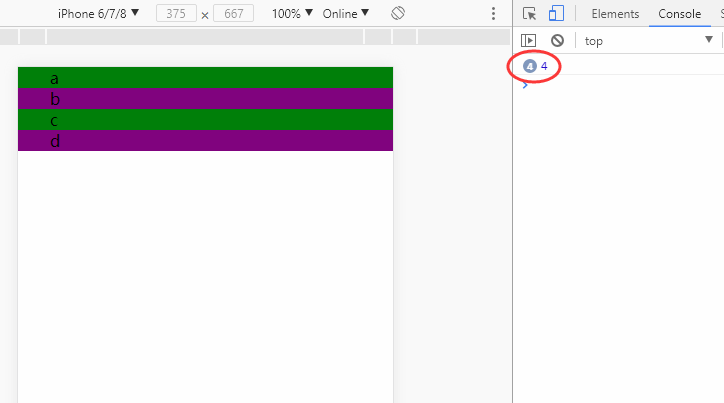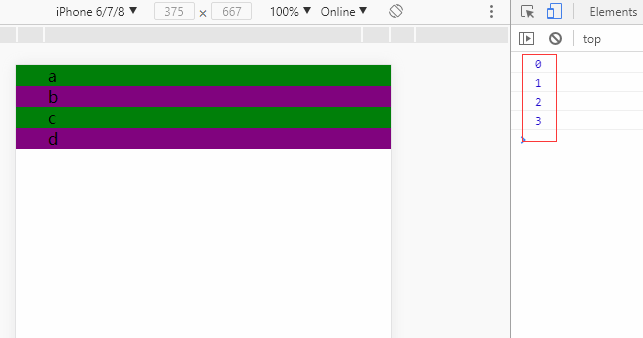# JavaScript学习笔记（9）- 立即执行函数

JavaScript学习笔记。本笔记是基于在腾讯课堂《Web前端开发之JavaScript精英课堂【渡一教育】》课程学习过程中记录的一些提纲和关键点。

### 立即执行函数的定义

``````(function test() {
var a = 123,
b = 234;
console.log(a + b); //357
}())

console.log(abc); //abc is not defined
``````

## 立即执行函数的书写形式

• (function () {}()) // W3C推荐写法
• (function () {})()

## 立即执行函数的执行

• 只有表达式才能被执行符号执行。
``````function test() {
var a = 123,
b = 234;
console.log(a + b); //357
}
test();
``````
• 能被执行符号执行的函数名称会被自动忽略，被执行符号执行的表达式就会变成立即执行函数。
``````var test = function () {
var a = 123,
b = 234;
console.log(a + b); //357
}()
console.log(test); //undefined
``````
• 变异的函数执行：通过在正常函数声明的前面添加`+``-``!`，后面添加`()`可变成立即执行函数。
``````[+/-/!] function test() {
var a = 123,
b = 234;
console.log(a + b); //357
}();
``````

``````function test(a, b, c, d) {
console.log(a + b + c + d);
}(1, 2, 3, 4);
``````

``````function test() {
var arr = [];
for(var i = 0; i < 10; i ++) {
arr[i] = function () {
document.write(i + " ");
}
}

return arr;
}

var myArr = test();
for(var j = 0; j < 10; j ++) {
myArr[j]();
}
``````

``````function test(){
var arr = [];
for(var i = 0; i < 10; i ++) {
(function (j){
arr[j] = function () {
document.write(j);
}
}(i))
}

return arr;
}

var myArr = test();
for(var j = 0; j < 10; j ++) {
myArr[j]();
}
``````

• 理解视觉上的误差：输出语句不是立即能够输出结果的，而是只有被执行后才能输出。
• 理解闭包产生的原因；
• 能够想到使用立即执行函数；
• 通过传参来解决数字在定义的时候能够保存并变现的问题。

``````<ul>
<li>a</li>
<li>b</li>
<li>c</li>
<li>d</li>
</ul>
``````

• 把所有`li`标签进行遍历存储；
• 通过点击事件来创建一个函数来输出索引；
• 利用`for`循环来循环输出。

``````<!DOCTYPE html>
<html>

<meta charset="utf-8" />
<meta http-equiv="X-UA-Compatible" content="IE=edge">
<title>Page Title</title>
<meta name="viewport" content="width=device-width, initial-scale=1">
<style>
body,
ul,
li {
margin: 0;
}

ul {
list-style: none;
}

li {
background-color: green;
text-indent: 2em;
}

li:nth-of-type(2n) {
background-color: purple;
}
</style>

<body>
<ul>
<li>a</li>
<li>b</li>
<li>c</li>
<li>d</li>
</ul>

<script>
var lis = document.getElementsByTagName('li');
for (var i = 0; i < lis.length; i++) {
lis[i].onclick = function () {
console.log(i);
}
}
</script>
</body>

</html>
````````````var lis = document.getElementsByTagName('li');
for (var i = 0; i < lis.length; i++) {
(function (j) {
lis[j].onclick = function () {
console.log(j);
}
}(i))
}
``````